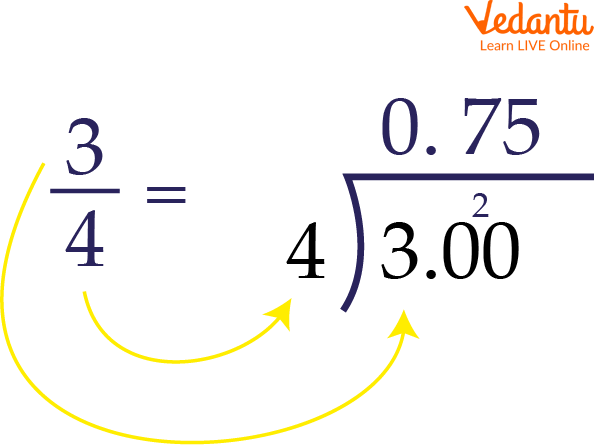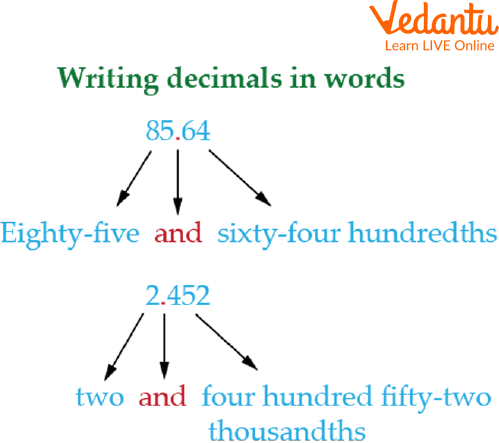Courses
Courses for Kids
Free study material
Free LIVE classes
More

# Introduction on How to write Fractions in Decimal FormLIVE
Join Vedantu’s FREE Mastercalss

## Diving Deep into the Decimals

We use decimals everyday while dealing with money, weight, length etc. Decimal numbers are used in situations where more precision is required than the whole numbers can provide. Numbers play a very vital role in Mathematics as well as our daily life. When we deal with fractions, sometimes their addition and subtraction get difficult to calculate. Hence there comes a need to convert fractions into the decimals or decimal numbers into their standard form. Let us study them in detail.Introduction to decimal numbers

## What is a Decimal?

A decimal is a number that means part of a whole. For example, the digit, or number, in front of a decimal represents a whole number. Let's say you have one apple; we would write that as 1.0. However, if you only have half of the apple, we can write that in decimal form by saying 0.5.

To express in decimal form, divide the numerator(top) by the denominator (bottom) like we simply do in the division.

For example, when we have a fraction say:

$\dfrac{2}{5}$ means $2 \div 5$, which is 0.4.How to convert fractions into decimals.

Sometimes fractions get converted into repeating decimals. A decimal in which one digit after the point is repeated infinitely is called a “Recurring decimal”.

For example, $\dfrac{2}{3}$ can be expressed in decimal form as 0.6666…. (where the number 6 after the point repeats infinitely). These are called recurring decimals. Repeating decimals or Recurring Decimals are often rounded to the nearest hundredth.

Here $\dfrac{2}{3}$ rounds to 0.67. Always remember,‘The first space to the right of the decimal point is its tenth place, the second space is the hundredth place, and the third is the thousand place, and so on..' Here, 0.666….. rounds to 0.67hundredths place.

0.2222….will round to 0.23 and so on.

## How to Write Decimals into Words?

Let us make the concept of writing a decimal into words easy. Here we will know how to write the decimal into a word using the example below-

85.64 will be written as Eighty-five and sixty-four hundredths.

Now let us see step by step how to convert a decimal into words-

Step 1- First of all read the digits to the left of the decimal point as a whole number.

Step 2- Say, ‘and’ for the decimal point.

Step3- Read the digits to the right of the decimal point as a whole number.

Step 4- Say the place of the last digit on the right.Decimal into words

For example, write the following decimal numbers 85.64 in words.

1. First, read the digits to the left of the decimal number as a whole number. Here it reads, ‘Eighty-five’.

2. Say ‘and’ for the decimal point.

3. Read the digit to the right of the decimal point as a whole number. Here it reads, sixty-four.

4. Now add the place of the last digit on the right i.e, hundredths.

So 85.64 will be read as ‘Eighty-five and sixty-four hundredths’.

Some more examples:

827.960 will be read as, Eight hundred twenty-seven and nine hundred sixty thousand.

0.002 will be two thousandths.

## Standard Form of a Decimal Number

Did you know that the distance between the earth and the sun is 150.24 million km which is a huge number to read? So we use millions or billions to make the number simple to read. That’s why we use a standard form to read, write and use the decimal number in many ways.

‘Any number that can be written as a decimal number between 1.0 and 10.00 multiplied by a power of 10 is said to be Standard Form of a Decimal number’.

Let us see some examples on the Standard form of a decimal number.

For example, $1,23,000$ can be written as $1.23 \times 10^5$.

If you observe carefully $1.23$ is a decimal number between $1.0$ to $10.00$.

Example- How to express 81,9000 in standard form,

Step1- Write the first digit, 8.

Step2- Add a decimal point after the first number and write the remaining non-zero number i.e, 8.19.

Step3- now count the number of digits after 8 . There are 5 digits after 8 .

So we write it as $8.19 \times 10^5$.

## How to Write the Expanded Form of a Decimal Number?

The expanded form of a decimal number is a way to write the number using the place value of each digit. To write the expanded form of a decimal number, you need to break it down into its individual digits and place them in their respective place value positions.

Here is an example of how to write the expanded form of the decimal number 5.678:

5.678 = 5 + (0.6) + (0.07) + (0.001)

In this example, the expanded form of the decimal number 5.678 is 5 + 0.6 + 0.07 + 0.001. The 5 is in the ones place, the 6 is in the tenths place, the 7 is in the hundredths place, and the 8 is in the thousandths place.

Another example, The expanded form of the decimal number 123.456

123.456 = 100 + 20 + 3 + (0.4) + (0.05) + (0.006)

In this example, the expanded form of the decimal number 123.456 is 100 + 20 + 3 + 0.4 + 0.05 + 0.006. The 1, 2 and 3 are in the hundredth place, the 4 is in the thousandths place, the 5 is in the ten-thousandths place and the 6 is in the hundred-thousandths place.

You can use this method to write the expanded form of any decimal number, just make sure to place each digit in the correct place value position.

## Solved Questions

Q 1. How do you express $\dfrac{4}{7}$ as a decimal?

Ans: To convert fraction $\dfrac{4}{7}$ into decimal, we divide 4 by 7.

$\dfrac{4}{7} = 4 \div 7$

=0.57

Hence, the decimal form of $\dfrac{4}{7}$ is 0.57.

Q 2. How do we write- 9.2, 74.5, 68.9 in words?

Ans: To write decimals into words,

9.2 = Nine and two tenths.

74.5 = Seventy four and five tenths.

68.9 = Sixty eight and nine tenths.

## Practice Questions

Q 1. How to write the following decimal number 700.14 into words?

Ans: Seven hundred and fourteen hundredths.

Q 2. Expand form of the decimal number 305 is?

Ans: 305=300+05

Q 3. How do we convert $\dfrac{2}{7}$ in decimal form?

Ans: $\dfrac{2}{7}$ =0.285.

## Summary

In this article, we learned about how Every day, we deal with decimals while dealing with money, weight, and length. When greater precision is required than whole numbers can give, decimal numbers are utilized converting fractions into decimals makes the operation easy and difficulty-free. The decimal form of a fraction, the standard form of a decimal number, and the expanded form of a decimal number are all very basic steps to learning and implementing mathematics. If you understand the concept thoroughly you can easily read and write decimal numbers.

Last updated date: 27th Sep 2023
Total views: 68.7k
Views today: 1.68k

## FAQs on Introduction on How to write Fractions in Decimal Form

1. What does ‘express as decimal’ mean?

Express in decimal means writing the decimal form of a fraction. For example,  ½ can be written as 0.5.

2. How do you write 9.5 in words?

9.5= Nine and five tenths.

3. Why do we need decimals in our daily life?

We use decimals everyday while dealing with money, weight, length etc. Decimal numbers are used in situations where more precision is required than the whole numbers can provide.